#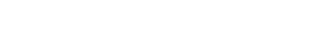When you do a computation with whole numbers, the Wolfram Language gives you an exact answer. It does the same with exact fractions.
1/2 + 1/3

N[1/2 + 1/3]

If there’s any decimal number in your input, the Wolfram Language will automatically give you an approximate answer.
The presence of a decimal number makes the result be approximate:
1.8/2 + 1/3

It’s enough just to have a decimal point at the end of a number:
1/2. + 1/3

The Wolfram Language can handle numbers of any size, at least so long as they fit in your computer’s memory.
Here’s 2 raised to the power 1000:
2^1000

Get a numerical approximation:
N[2^1000]

Enter a number in scientific notation:
2.7*^6

Commonly used numbers like π (pi) are built into the Wolfram Language.
Get a numerical approximation to π:
N[Pi]

The Wolfram Language can compute to arbitrary precision, so for example it can find millions of digits of π if you want them.
Compute 250 digits of π:
N[Pi, 250]

There are many functions in the Wolfram Language that handle integers (whole numbers). There are also many functions that handle real numbersapproximate numbers with decimals. An example is RandomReal, which gives random real numbers.
Generate a random real number in the range 0 to 10:
RandomReal

Generate 5 random real numbers:
Table[RandomReal, 5]

An alternative way to ask for 5 random real numbers:
RandomReal[10, 5]

RandomReal[{20, 30}, 5]

The Wolfram Language has a huge range of mathematical functions built in, from basic to very sophisticated.
Find the 100th prime number:
Prime

Find the millionth prime number:
Prime

Plot the first 50 primes:
ListPlot[Table[Prime[n], {n, 50}]]

Three functions common in many practical situations are Sqrt (square root), Log10 (logarithm to base 10) and Log (natural logarithm).
The square root of 16 is 4:
Sqrt

If you don’t ask for a numerical approximation, you’ll get an exact formula:
Sqrt

N gives a numerical approximation:
N[Sqrt]

Logarithms are often useful when you’re dealing with numbers that have a wide range of sizes. Let’s plot the masses of the planets. With ListPlot one can’t tell anything about the planets before Jupiter. But ListLogPlot shows the relative sizes much more clearly.
Make an ordinary ListPlot of the masses of the planets:
ListPlot[EntityClass["Planet", All]["Mass"]]

Make a log plot:
ListLogPlot[EntityClass["Planet", All]["Mass"]]

There are a few more functions that show up frequently in general programming. First, there’s the almost trivial function Abs, that finds the absolute value, or positive part, of a number.
Abs effectively just drops minus signs:
{Abs, Abs[-3]}

Next there’s Round, which rounds to the nearest whole number.
Round rounds to the nearest whole number:
{Round[3.2], Round[3.4], Round[3.6], Round[3.9]}

Another function that’s very useful is Mod. Let’s say you’re counting up minutes in an hour. When you reach 60, you’ll want to start again from 0. That’s what Mod lets you do.
Compute a sequence of numbers mod 60:
{Mod[50, 60], Mod[55, 60], Mod[60, 60], Mod[65, 60], Mod[70, 60]}

 N[expr] numerical approximation N[expr,n] numerical approximation to n-digit precision Pi the number π (pi) ≃ 3.14 Sqrt[x] square root Log10[x] logarithm to base 10 Log[x] natural logarithm (ln) Abs[x] absolute value (drop minus signs) Round[x] round to nearest integer Prime[n] nth prime number Mod[x,n] modulo (“clock arithmetic”) RandomReal[max] random real number between 0 and max RandomReal[max,n] list of n random real numbers ListLogPlot[data] plot on a logarithmic scale
23.1Find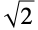to 500-digit precision. »
Expected output:
 Out[]=Answer & check your solution
23.2Generate 10 random real numbers between 0 and 1. »
Sample expected output:
 Out[]=Answer & check your solution
23.3Make a plot of 200 points with random real x and y coordinates between 0 and 1. »
Sample expected output:
 Out[]=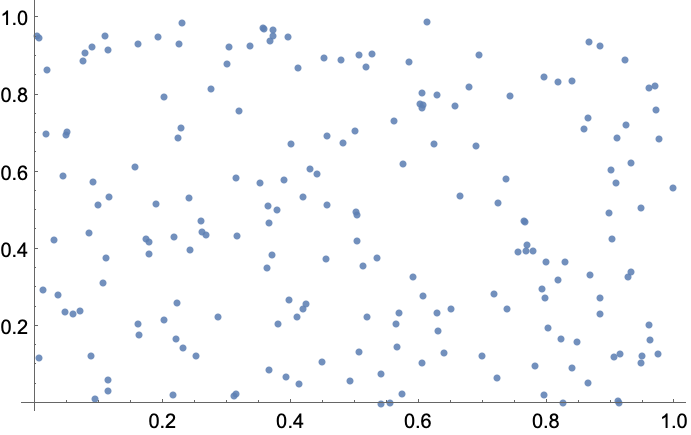Answer & check your solution
23.4Create a random walk using AnglePath and 1000 random real numbers between 0 and 2π»
Sample expected output:
 Out[]=Answer & check your solution
23.5Make a table of Mod[n^2, 10] for n from 0 to 30. »
Expected output:
 Out[]=Answer & check your solution
23.6Make a line plot of Mod[n^n, 10] for n from 1 to 100. »
Expected output:
 Out[]=Answer & check your solution
23.7Make a table of the first 10 powers of π, rounded to integers. »
Expected output:
 Out[]=Answer & check your solution
23.8Make a graph by connecting n with Mod[n^2, 100] for n from 0 to 99. »
Expected output:
 Out[]=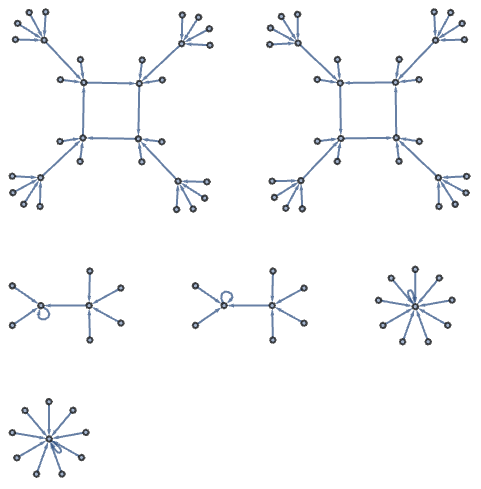Answer & check your solution
23.9Generate graphics of 50 circles with random real coordinates 0 to 10, random real radii from 0 to 2, and random colors. »
Sample expected output:
 Out[]=Answer & check your solution
23.10Make a plot of the nth prime divided by n*log(n), for n from 2 to 1000. »
Expected output:
 Out[]=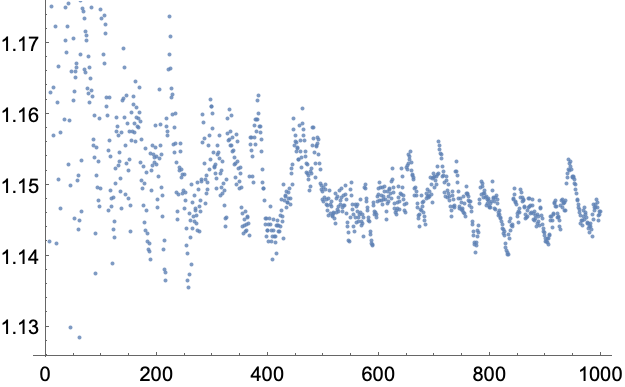Answer & check your solution
23.11Make a line plot of the differences between successive primes up to 100. »
Expected output:
 Out[]=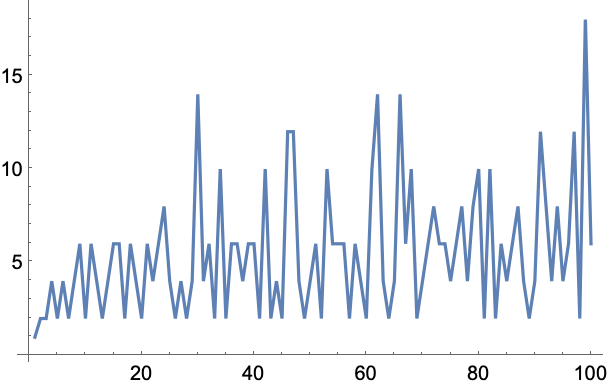Answer & check your solution
23.12Generate a sequence of 20 middle C notes with random durations between 0 and 0.5 seconds. »
Sample expected output:
 Out[]=Answer & check your solution
23.13Make an array plot of Mod[i, j] for i and j up to 50. »
Expected output:
 Out[]=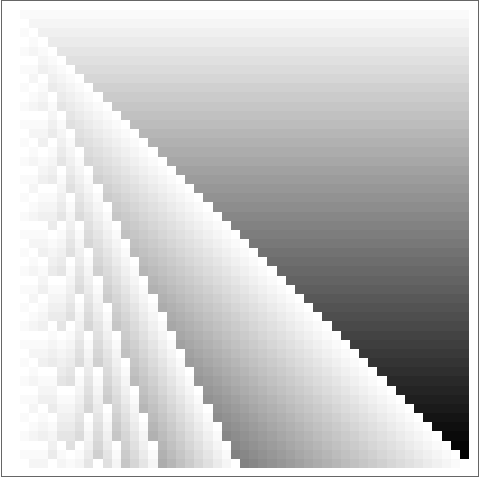Answer & check your solution
Expected output:
 Out[]=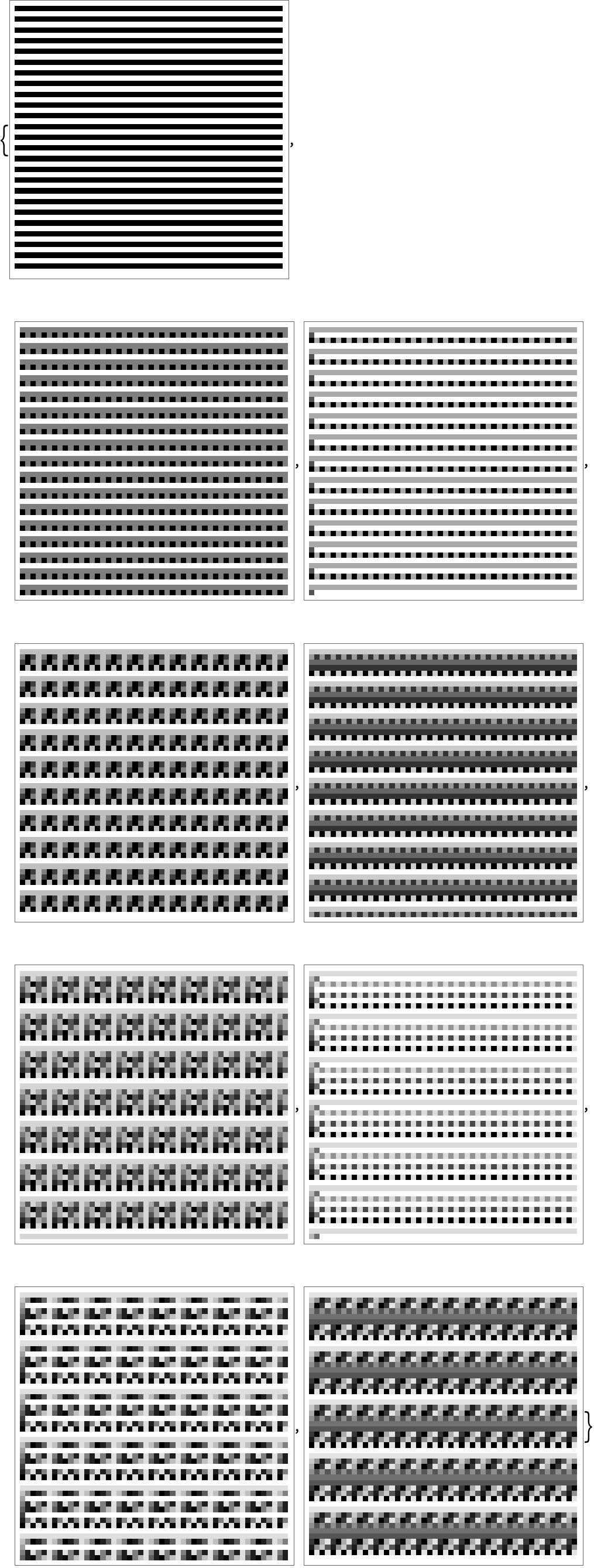Answer & check your solution
+23.1Use Round to compute the fractional part of π to 50 digits. »
Expected output:
 Out[]=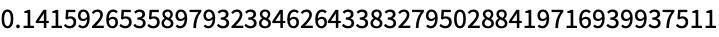Answer & check your solution
+23.2Find the sum of the first 1000 prime numbers. »
Expected output:
 Out[]=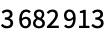Answer & check your solution
+23.3Make a list of the first 100 primes modulo 4. »
Expected output:
 Out[]=Answer & check your solution
+23.4Make a list of the first 10000 primes modulo 4, multiply them by 90° and create an angle path from them. »
Expected output:
 Out[]=Answer & check your solution
What are examples of mathematical functions in the Wolfram Language?
From standard school math, ones like Sin, Cos, ArcTan, Exp, as well as GCD, Factorial, Fibonacci. From physics and engineering and higher math, ones like Gamma (“gamma function”), BesselJ (“Bessel function”), EllipticK (“elliptic integral”), Zeta (“Riemann zeta function”), PrimePi, EulerPhi. From statistics, ones like Erf, NormalDistribution, ChiSquareDistribution. Hundreds of functions altogether.
What is the precision of a number?
It’s the total number of decimal digits quoted in the number. N[100/3, 5] gives 33.333, which has 5 digits of precision. The number 100/3 is exact; N[100/3, 5] approximates it to 5-digit precision.
What does the at the end of each line in a long number mean?
It’s there to show that the number continues onto the next linelike a hyphen in text.
Can I work with numbers in bases other than 10?
Yes. Enter a number in base 16 as 16^^ffa5. Find digits using IntegerDigits[655, 16].
Can the Wolfram Language handle complex numbers?
Of course. The symbol I (capital “i”) represents the square root of 1.
Why does N[1.5/7, 100] not give me a 100-digit result?
Because 1.5 is an approximate number with much less than 100-digit precision. N[15/70, 100] will for example give a 100-digit-precision number.
How can I control the display of numbers?
N[expr, n] computes to n-digit precision. NumberForm[expr, m] displays m digits. DecimalForm[expr] avoids scientific notation. PercentForm[expr] displays as a percentage.
• The Wolfram Language does “arbitrary-precision computation”, meaning that it can keep as many digits in a number as you want.
• When you generate a number with a certain precision using N, the Wolfram Language will automatically keep track of how that precision is affected by computationsso you don’t have to do your own numerical analysis of roundoff errors.
• If you type a number like 1.5, it’s assumed to be at the native “machine precision” of numbers on your computer (usually about 16 digits, though only 6 are usually displayed). Use 1.5 `100 to specify 100-digit precision.
• With exact input (like 4 or 2/3 or Pi), the Wolfram Language always tries to give exact output. But if the input contains an approximate number (like 2.3), or if you use N, it’ll use numerical approximation.
• Numerical approximation is often crucial in making large-scale computations feasible.
• PrimeQ tests if a number is prime (see Section 28). FactorInteger finds the factors of an integer.
• RandomReal can give numbers that aren’t just uniformly distributed. For example, gives normally distributed numbers.
• Round rounds to the nearest integer (up or down); Floor always rounds down; Ceiling always rounds up.
• RealDigits is the analog of IntegerDigits for real numbers.
• Around[x, dx] (displayed as x±dx) lets you work with “numbers with errors”, as can arise from real-world measurements.